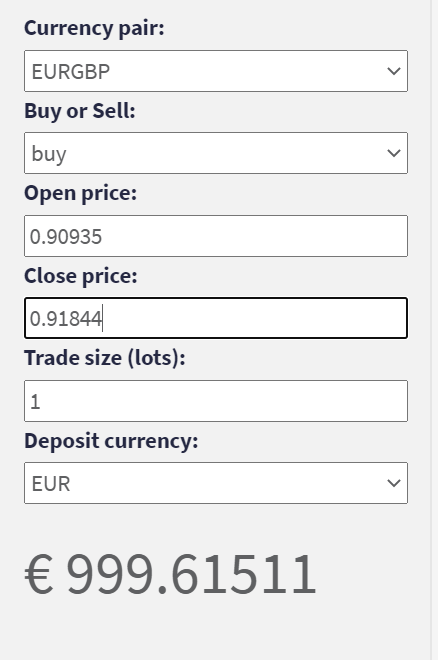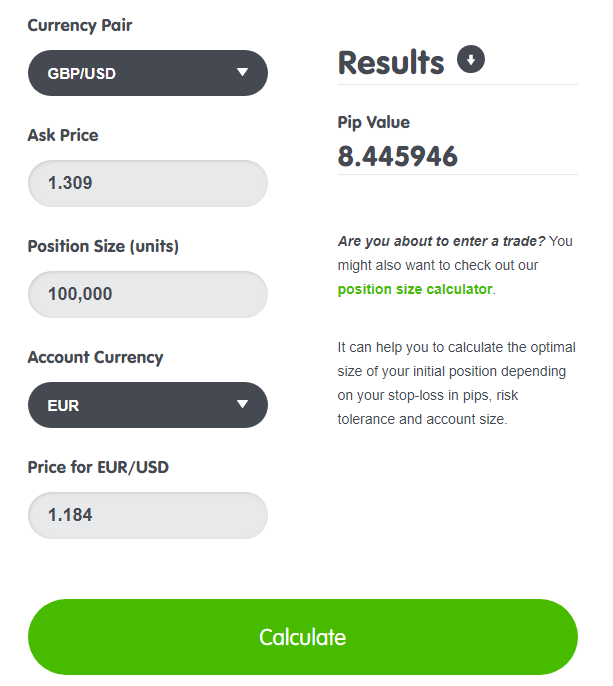# The profit/loss formula looks wrong to me

Ok, I know JPY and GBP have different pip digits and this affect the formula, but the formula itself looks weird to me… look at these images:

Both examples have the same identical inputs, so, in the same formula, they should give the same output. I know EURGBP won’t ever get that high, but theorically it’s possible, pip digits is something we decided and I don’t see any reason why they should affect profit and losses

The most confusing part is: Is there an explaination of why, in EURGBP even if the price increases of just 1%, the profit becomes more than the capital itself…
If the price dropped, and become 98 instead than 101, I would have lost more than my whole capital, And that looks impossible without leverage, until the price drops to 0 (so the currency loose all its value).

What is the result from this calculator if you enter the actual current prices for entry and add 1% for the exits?This happens, I know that this looks right, but I can’t see the reason that if the price is like 100, a 1% increase, doesn’t give the same results as this one

Hi, and welcome to this forum.

I think I can shed some light on the calculations you posted.

In your second post, you used an actual EUR/GBP price for your entry, and you correctly calculated an exit price based on a 1% price rise.

Entry (long) at EUR/GBP = 0.90935, Exit at EUR/GBP = 0.91844

Your profit was 0.91844 - 0.90935 = 0.00909 = 90.9 pips

Using the Babypips Pip Value Calculator, we calculate that 1 pip = 10.996866 EUR per standard lot.

[10.996866 EUR / pip / lot] x 90.9 pips x 1 lot = 999.61511 EUR

which agrees with the result you displayed in your second post.

If we apply exactly this same methodology to the two examples given in your first post, your two examples will produce results that agree with each other, just as you expected they would. However, those results do not agree with either of the results you displayed in your first post. Let’s go through it.

But, first –

Actually, pip-values do affect these calculations, as the following examples will illustrate.

Starting with your EUR/JPY example –

Entry (long) at EUR/JPY = 100.00, Exit at EUR/JPY = 101.00

Your profit was 101.00 - 100.00 = 1.00 = 100 pips

Using the Babypips Pip Value Calculator, we calculate that 1 pip = 10 EUR (exactly) per standard lot.

[10 EUR / pip / lot] x 100 pips x 1 lot = 1,000 EUR

Now, let’s apply the same methodology to your EUR/GBP example –

Entry (long) at EUR/GBP = 100.0000, Exit at EUR/GBP = 101.0000

Your profit was 101.0000 - 100.0000 = 1.0000 = 10,000 pips

Using the Babypips Pip Value Calculator, we calculate that 1 pip = 0.1 EUR (exactly) per standard lot.

Therefore, you profit was

[0.1 EUR / pip / lot] x 10,000 pips x 1 lot = 1,000 EUR

so the EUR/GBP example yields the same result as the EUR/JPY example.

As for the results in your first post, I have no idea how your calculator arrived at those figures.

One point needs to be made here:

Your calculator obviously used your entry prices to determine pip values. In order to get my example (above) to agree with the result in your second post, I plugged your entry price (0.90935) into the Babypips Pip Value Calculator, as well.

This is technically incorrect. When a trade is closed, P/L is calculated based on the pip value at the closing price of the trade, not the entry price.

Last point –

Always pay attention to the difference between the yen and all other major currencies. One yen is worth about 1/100 of what each of the other currencies is worth. Accordingly, all the yen metrics are multiplied by 100, in order to make calculations versus the other currencies possible.

That’s why one pip in any yen pair is represented by the second digit to the right of the decimal point, whereas in all non-yen pairs one pip is represented by the fourth digit to the right of the decimal point.

However, this does not explain the weird results you got in your first post. As I said above, I have no idea how your calculator got those results.

3 Likes

I really have to thank you for your answerI think I understand, it looks like that calculator might be using a fixed pip value or something like that giving me those impossible results. (I was using the first result on google for profit forex calculator)

I know that the yen is 100 times normal currencies, but theorically, also eurgbp coud become that high, so decimal place should be changed at that point.
All this poblem begins from the fact that I needed a formula to calculate lot size, knowing entry price and SL. I thought I was doing someething wrong, cause results didn’t match…
It should be something like this: profit/loss = (Sell price - Buy price)xPip value per lot(on close price)x Lots x lot value . I was just confused by the fact that pip value changes if price changes, changing the symbol exchange rate, so I couldn’t understand how any of those formulas could take count of that, without using integrals… Using the close price solve that, right?

I don’t know where you found that calculator, or how it works (or fails to work).

I do know that Babypips offers a selection of free forex calculators that do work. Click the Tools tab at the top of this page, and get acquainted with those calculators. Used correctly, they are accurate and reliable.

If the pound sterling were to crash and burn to the point of being equal in value to one yen, then probably adjustments would have to be made in the way GBP-pairs were quoted. But, this is idle speculation.

While you are trying to learn the basics of this market, don’t clutter your brain with extreme conjectures about things that are never going to happen.

Let’s forget about strange calculators that give weird results, and let’s forget about science-fiction scenarios like GBP crashing down to the value of one yen.

I can give you a formula that answers your question, and I will do so in my next post.

In the examples we covered, using exit prices, instead of entry prices, to determine the pip values would have made a small correction to the results calculated – but, would not have corrected the problems caused by (1) using a weird calculator, and (2) using a bizarre price of EUR/GBP = 100.0000

• Pip values depend on the currency in question: A JPY-pip is not equal to a EUR-pip.

• Pip values also depend on position size: One yen-pip per unit of currency does not equal one yen-pip per mini-lot, or one yen-pip per standard lot.

• Finally, P/L (profit or loss in a particular trade) depends on (1) the currencies involved, (2) position size, and (3) the number of pips gained or lost in the trade.

All this will become clearer when I give you that formula I promised.

1 Like

Here’s a simple formula for P/L in which pips and pip values are implied, but you don’t actually have to deal with them.

Suppose you are trading currency pair A/B in which A is the base currency, and B is the quote currency.

And suppose that your account currency is C.

You enter a trade (LONG) at A/B = Entry price, and you exit your trade at A/B = Exit price.

You earned a profit (per unit of currency traded) equal to Exit price - Entry price. This profit is stated in units of B, the quote currency.

But, you want P/L for your full trade, not for one unit of currency. And you want it stated in C, your account currency.

We can now write –

P/L = (Exit price - Entry price) x position size in units ÷ price of C/B at time of exit

How did we get around dealing directly with pips and pip values?

Well, the calculated price difference between entry and exit is, in fact, the pip gain in decimal form. And factoring in the price of C/B converts B-pips to C-pips.

Let’s use actual numbers to illustrate how easy it is to use this formula.

Suppose you are trading GBP/USD in your EUR-denominated account. In other words, referring to the generalized formula above, A=GBP, B=USD, and C=EUR.

Let’s say you enter GBP/USD (LONG) at 1.3000, and you exit GBP/USD at 1.3090.

Your position size is 100,000 units (one standard lot).

At the time of your exit, the ASK price for EUR/USD was 1.1840

P/L = (1.3090 - 1.3000) x 100,000 ÷ 1.1840 = 760.13513 EUR

Can we verify this result using pip values? Absolutely.

Let’s start with the Babypips Pip Value Calculator. We enter the following metrics into the Calculator –

• Currency pair: GBP/USD
• Ask Price (for GBP/USD): 1.3090 at exit
• Position size: 100000
• Account currency: EUR
• Price for EUR/USD: 1.1840 at exit

Here’s how it looks –The Pip Value Calculator tells us that one pip (per 100,000 units traded) is worth 8.445946 EUR

Thanks a lot